# IB DP Physics: HL复习笔记10.2.2 Potential Energy Calculations

### Potential Energy Calculations

#### Electric Potential Energy of Two Point Charges

• The electric potential energy Ep at point in an electric field is defined as:

The work done in bringing a charge from infinity to that point

• The electric potential energy of a pair of point charges Q1and Q2 is defined by: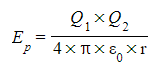Where:
• Ep = electric potential energy (J)
• r = separation of the charges Q1 and Q2 (m)
• ε0 = permittivity of free space (F m-1)
• The potential energy equation is defined by the work done in moving point charge Q2 from infinity towards a point charge Q1.
• The work done is equal to: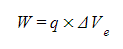Where:
• W = work done (J)
• V = electric potential due to a point charge (V)
• Q = Charge producing the potential (C)
• This equation is relevant to calculate the work done due on a charge in a uniform field
• Unlike the electric potential, the potential energy will always be positive
• Recall that at infinity, V = 0 therefore Ep = 0
• It is more useful to find the change in potential energy, for example, as one charge moves away from another
• The change in potential energy from a charge Q1 at a distance r1 from the centre of charge Q2 to a distance r2 is equal to: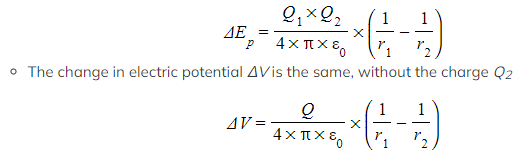There is another similarity with gravitational potential, as both equations are very similar to the change in gravitational potential between two points near a point mass

#### Worked Example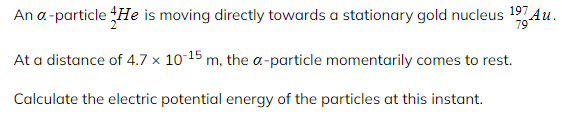Step 1: Write down the known quantities

• Distance, r = 4.7 × 10-15 m
• The charge of one proton, q = +1.60 × 10-19 C

An alpha particle (Helium nucleus) has 2 protons

• Charge of alpha particle, Q1 = 2 × 1.60 × 10-19 = +3.2 × 10-19 C

The gold nucleus has 79 protons

• Charge of gold nucleus, Q2 = 79 × 1.60 × 10-19 = +1.264 × 10-17 C

Step 2: Write down the equation for electric potential energy

####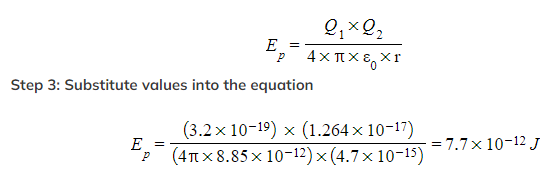Gravitational Potential Energy Between Two Point Masses

• The gravitational potential energy (G.P.E) at point in a gravitational field is defined as:

The work done in bringing a mass from infinity to that point

• The equation for G.P.E of two point masses m and M at a distance r is:
•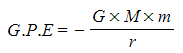G.P.E is calculated using mgh, but recall that at infinity, g = 0 and therefore G.P.E = 0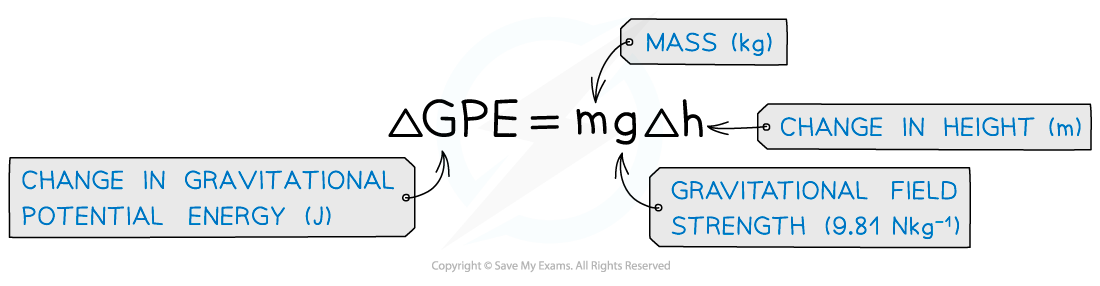• It is more useful to find the change in G.P.E for example for a satellite which is lifted into space from the Earth’s surface
• The change in G.P.E from for an object of mass m at a distance r1 from the centre of mass M, to a distance of r2 further away is: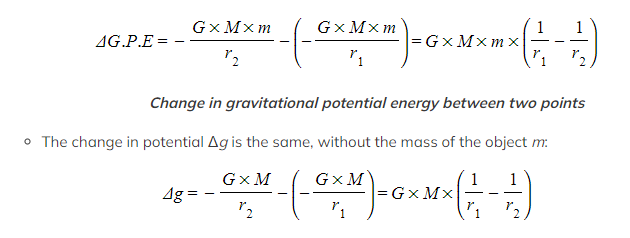Change in gravitational potential between two points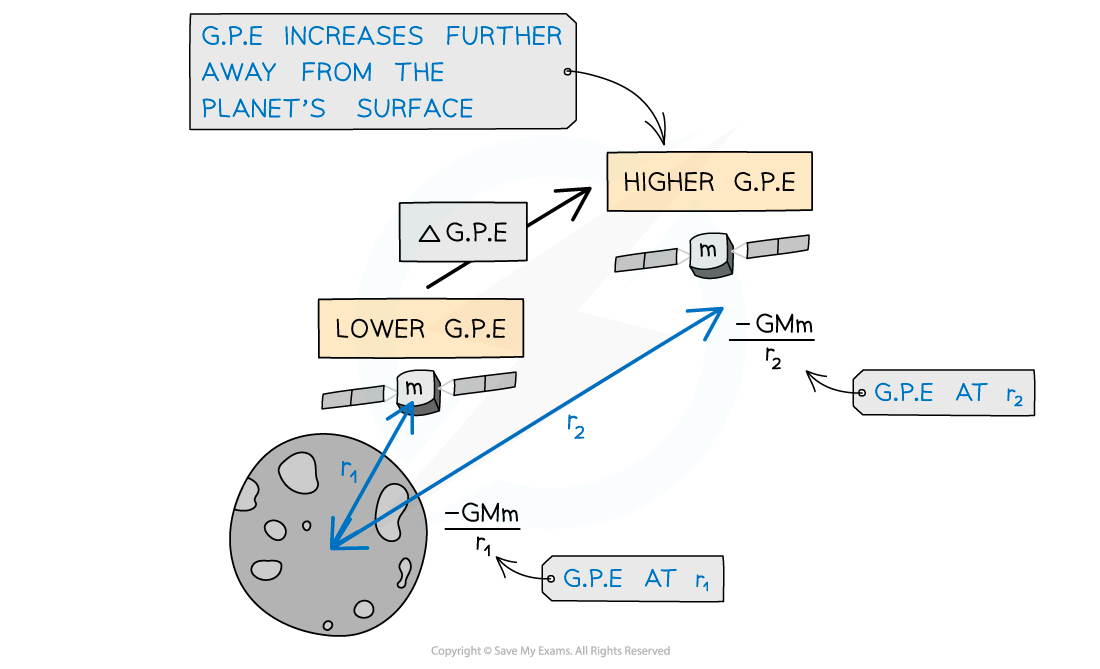Gravitational potential energy increases as a satellite leaves the surface of the Moon

#### Worked Example

Calculate the difference in potential energy when a satellite of mass 1450 kg when it is moved from a distant orbit of 980 km above Earth’s surface to a closer orbit of 480 km above the Earth’s surface. Assume the Earth’s mass to be 5.97 x 1024 kg and the radius of the Earth to be 6.38 x 106 m.

Step 1: Write down the known quantities
• Initial distance of orbit above Earth’s surface: 980 km
• Final distance of orbit above Earth’s surface: 480 km
• Mass of the satellite: m = 1450 kg
• Earth’s mass: M = 5.97 x 1024 kg
• Radius of the Earth: 6.38 x 106 m
Step 2: Write down the equation for change in gravitational potential energy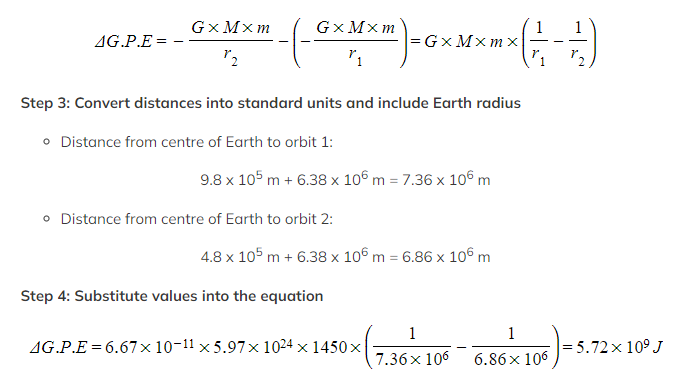• The difference in gravitational potential energy between the two orbits is: 5.72 x 109 J